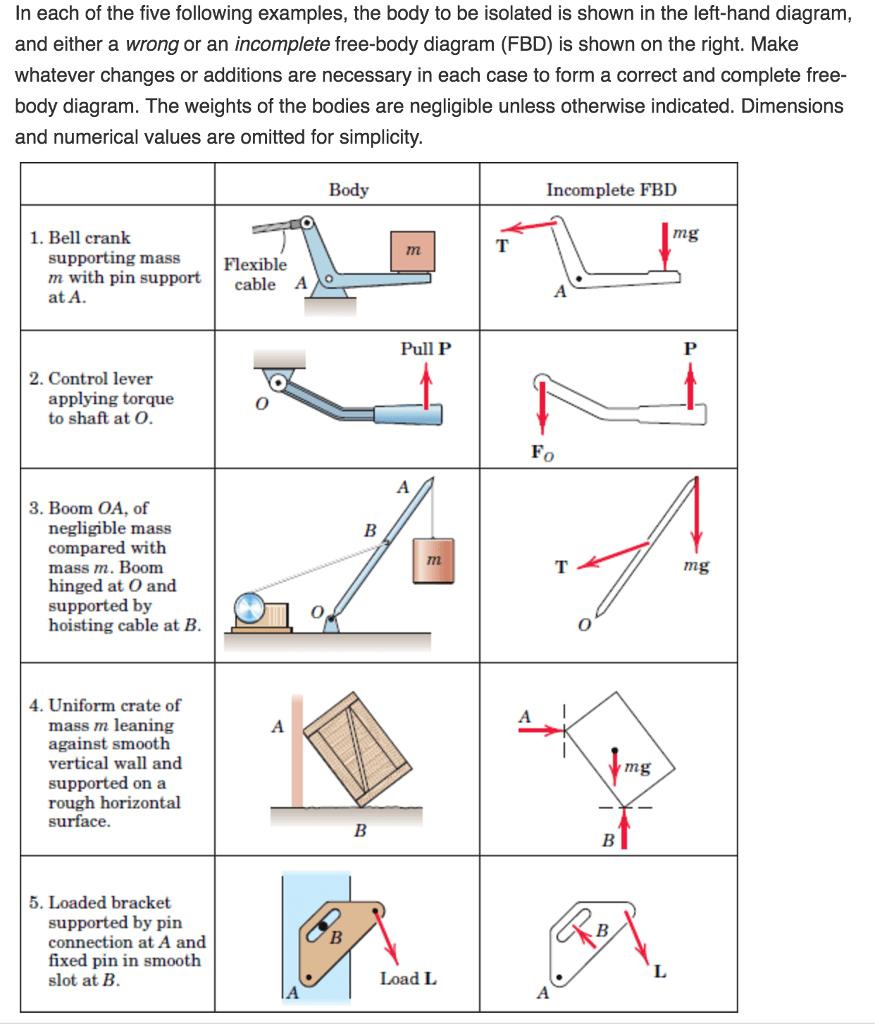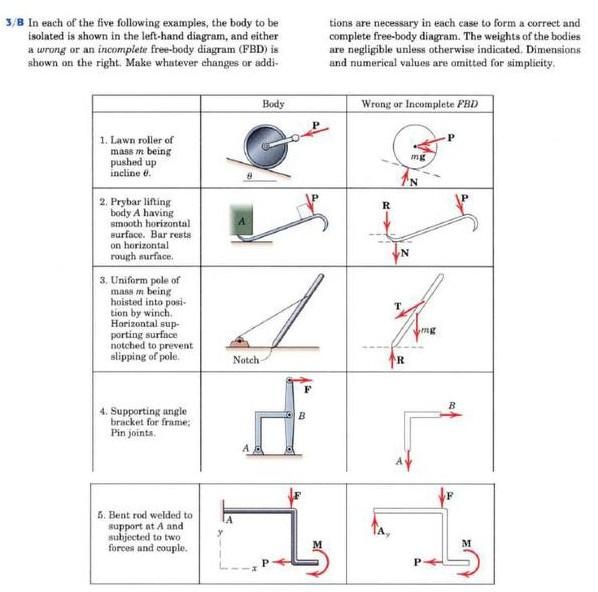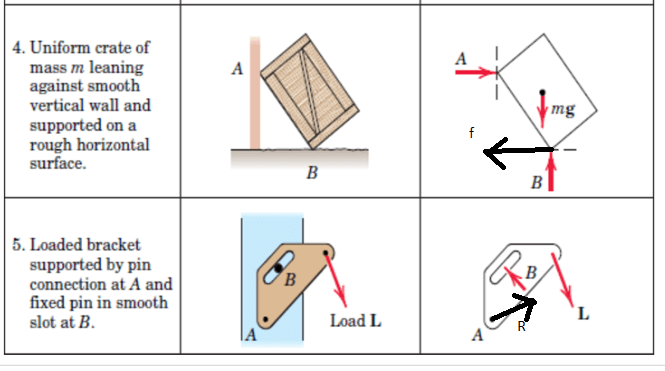# In each of the five following examples, the body to be isolated is shown in the left-hand diagram, and either a wrong or an incomplete free-body diagram (FBD) is shown on the right. Make whatever changes or additions are necessary in each case to form a correct and complete free- body diagram. The weights of the bodies are negligible unless otherwise indicated. Dimensions and numerical values are omitted for simplicity  m-fbd-is-shown-on-the-right-make-whatever-chan

Question-AnswerCategory: Engineering MechanicsIn each of the five following examples, the body to be isolated is shown in the left-hand diagram, and either a wrong or an incomplete free-body diagram (FBD) is shown on the right. Make whatever changes or additions are necessary in each case to form a correct and complete free- body diagram. The weights of the bodies are negligible unless otherwise indicated. Dimensions and numerical values are omitted for simplicity  m-fbd-is-shown-on-the-right-make-whatever-chan

In each of the five following examples, the body to be isolated is shown in the left-hand diagram, and either a wrong or an incomplete free-body diagram (FBD) is shown on the right. Make whatever changes or additions are necessary in each case to form a correct and complete free- body diagram. The weights of the bodies are negligible unless otherwise indicated. Dimensions and numerical values are omitted for simplicityQuestion 2The following are the updated FBD’sQuestion 2Question 2